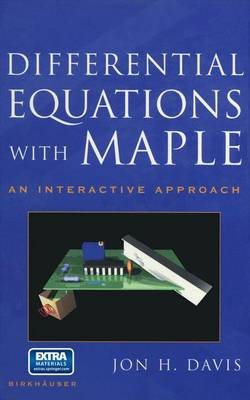•Differential Equations with Maple: An Interactive Approach (Hardback)

(author)
£69.99
Hardback 411 Pages / Published: 21/12/2000
• We can order this

Usually dispatched within 3 weeks

Differential equations is a subject of wide applicability, and knowledge of dif- Differential equations is a subject of wide applicability, and knowledge of dif- ferential ferential equations equations topics topics permeates permeates all all areas areas of of study study in in engineering engineering and and applied applied mathematics. mathematics. Some Some differential differential equations equations are are susceptible susceptible to to analytic analytic means means of of so- so- lution, lution, while while others others require require the the generation generation of of numerical numerical solution solution trajectories trajectories to to see see the the behavior behavior of of the the system system under under study. study. For For both both situations, situations, the the software software package package Maple Maple can can be be used used to to advantage. advantage. To To the the student student Making Making effective effective use use of of differential differential equations equations requires requires facility facility in in recognizing recognizing and and solving solving standard standard "tractable" "tractable" problems, problems, as as well well as as having having the the background background in in the the subject subject to to make make use use of of tools tools for for dealing dealing with with situations situations that that are are not not amenable amenable to to simple simple analytic analytic approaches. approaches.

Publisher: Birkhauser Boston Inc
ISBN: 9780817641818
Number of pages: 411
Weight: 805 g
Dimensions: 235 x 155 x 23 mm
Edition: 2001 ed.

MEDIA REVIEWS

"A very novel approach to the teaching/learning of all basic aspects of differential equations. ... The book, therefore, provides an introduction to MAPLE as well as standard material on differential equations written in a friendly style." -Aslib Book Guide

"The course in differential equations starts with fairly well-explained examples from different fields of application. The theory is presented in a student-friendly, understandable form.... The problems presented at the end of each chapter contain material for using the theory or the tools presented in the frame of MAPLE. ... The textbook is recommended for those who start solving differential equation problems by MAPLE and also for those who need an introduction in (mainly ordinary) differential equations." -Applications of Mathematics